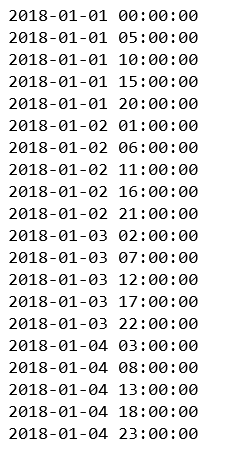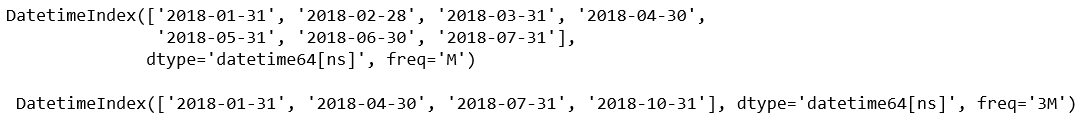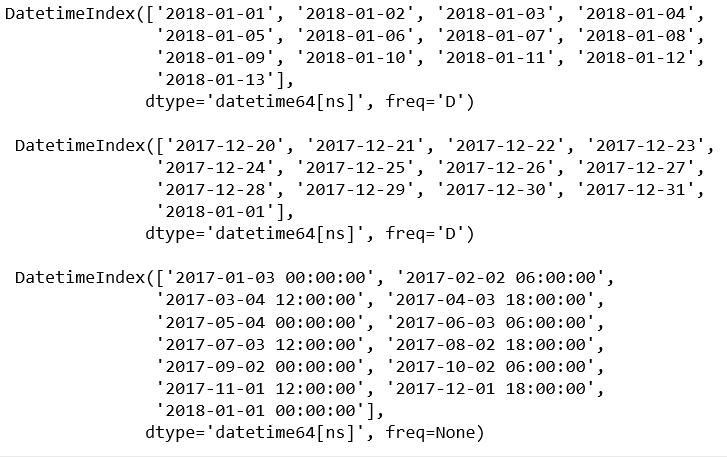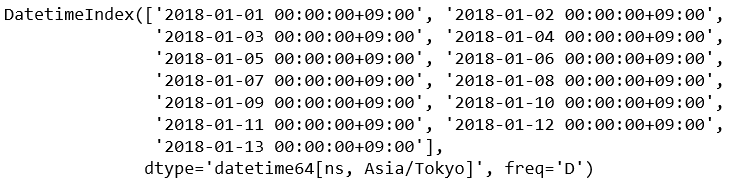Open in App
Not now

# Python | pandas.date_range() method

• Last Updated : 17 Dec, 2018

Python is a great language for doing data analysis, primarily because of the fantastic ecosystem of data-centric python packages. Pandas is one of those packages and makes importing and analyzing data much easier.

`pandas.date_range()` is one of the general functions in Pandas which is used to return a fixed frequency DatetimeIndex.

Syntax: pandas.date_range(start=None, end=None, periods=None, freq=None, tz=None, normalize=False, name=None, closed=None, **kwargs)

Parameters:
start : Left bound for generating dates.
end : Right bound for generating dates.
periods : Number of periods to generate.
freq : Frequency strings can have multiples, e.g. ‘5H’. See here for a list of frequency aliases.
tz : Time zone name for returning localized DatetimeIndex. By default, the resulting DatetimeIndex is timezone-naive.
normalize : Normalize start/end dates to midnight before generating date range.
name : Name of the resulting DatetimeIndex.
closed : Make the interval closed with respect to the given frequency to the ‘left’, ‘right’, or both sides (None, the default).

Returns: DatetimeIndex

Code #1:

 `# importing pandas as pd``import` `pandas as pd`` ` `per1 ``=` `pd.date_range(start ``=``'1-1-2018'``, ``         ``end ``=``'1-05-2018'``, freq ``=``'5H'``)`` ` `for` `val ``in` `per1:``    ``print``(val)`

Output:Code #2:

 `# importing pandas as pd``import` `pandas as pd`` ` `dRan1 ``=` `pd.date_range(start ``=``'1-1-2018'``,``           ``end ``=``'8-01-2018'``, freq ``=``'M'``)`` ` `dRan2 ``=` `pd.date_range(start ``=``'1-1-2018'``, ``         ``end ``=``'11-01-2018'``, freq ``=``'3M'``)`` ` `print``(dRan1, ``'\n\n'``, dRan2)`

Output:Code #3:

 `# importing pandas as pd``import` `pandas as pd`` ` `# Specify start and periods, the number of periods (days).``dRan1 ``=` `pd.date_range(start ``=``'1-1-2018'``, periods ``=` `13``)`` ` `# Specify end and periods, the number of periods (days).``dRan2 ``=` `pd.date_range(end ``=``'1-1-2018'``, periods ``=` `13``)`` ` `# Specify start, end, and periods; the frequency ``# is generated automatically (linearly spaced).``dRan3 ``=` `pd.date_range(start ``=``'01-03-2017'``, ``            ``end ``=``'1-1-2018'``, periods ``=` `13``)`` ` `print``(dRan1, ``"\n\n"``, dRan2, ``'\n\n'``, dRan3)`

Output:Code #4:

 `# importing pandas as pd``import` `pandas as pd`` ` `# Specify start and periods, the number of periods (days).``dRan1 ``=` `pd.date_range(start ``=``'1-1-2018'``, ``       ``periods ``=` `13``, tz ``=``'Asia / Tokyo'``)`` ` `dRan1`

Output:My Personal Notes arrow_drop_up